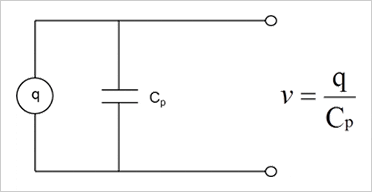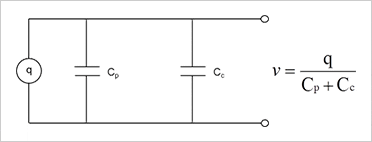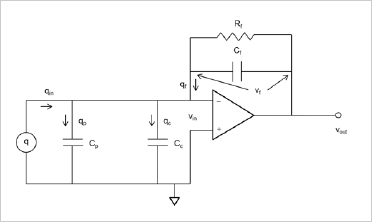Question

I have one of your piezoelectric accelerometers that I plan to use in a vibration test on a shaker. I'm told I have to use a charge amplifier with the accelerometer. What is a charge amplifier and why do I need it? Can't I just use a voltage amplifier?

Up until the mid-1960s, piezoelectric accelerometers were calibrated in voltage units (mV/g) and voltage amplifiers were used to signal condition these accelerometers. However, there were many drawbacks to this technology scheme. Charge amplifiers were commercially viable by the mid-1960s and thereafter piezoelectric accelerometers were calibrated in charge units (pC/g), with the intent that a charge amplifier be used to signal condition the sensor. Let's examine this in more detail to see why.

Voltage amplifier basics:

A piezoelectric accelerometer can be modeled as a charge generator and a capacitor, as shown in Figure 1 below:Figure 1

Cp is the capacitance of the piezoelectric sensing element itself. The relationship between charge and voltage on a capacitor, q = CV, is used to determine the voltage at the terminals of the accelerometer. However, what happens when a length of cable is added? What is the voltage at the end of some length of cable? Figure 2 models this situation, where the lump sum of the distributed cable capacitance is represented by Cc.Figure 2

Clearly the voltage value has changed (decreased) because the capacitance has changed (increased). If different lengths of cables are used, different values of voltage will result. This is why early model piezoelectric accelerometers were calibrated with the stipulation that a certain cable capacitance was used. See Figure 3 as an example. This is a calibration report for an Endevco model 2213 (obsolete long ago) piezoelectric accelerometer (from 1958!). Note the footnote that 100 pF (100 ÂµÂµF) of cable capacitance has been added.Figure 3

Using voltage (and by extension voltage amplifiers) is clearly an inconvenient, if not difficult or impossible, situation. What can we do about this?

Charge amplifier basics:

First, we can make the observation that the amount of charge generated does not change, regardless of the amount of cable capacitance added (in contrast to the voltage at the terminals). So what we really need is a "charge sensing" circuit, not a voltage sensing circuit. This is the basis of the "charge converter" circuit (or more broadly, the charge amplifier). Let's take a closer look.

Figure 4 is our piezoelectric accelerometer model with cable capacitance attached, but with a charge converter circuit connected, using an operation amplifier (or op amp). Individual charge flows and node voltages have been identified to facilitate further analysis.Figure 4

Our analysis goal is to end up with a gain equation in terms of vout/qin. In the process, we will gain some insight into how a charge amplifier deals with the problem of changing cable capacitance.

First, let's sum all the charge flows:

qin = qp + qc + qf

Because of the relationship q = CV, we can rewrite the above as:

qin = vinCp + vinCc + vfCf

or

qin = vin(Cp + Cc) + vfCf

We know, however, that vin = 0, because of the virtual short across the input terminals of the op amp (assume an ideal op amp). So the equation above simplifies to:

qin = vfCf

Rearranging, we have:

vf = qin/Cf

Again, because of the virtual short across the terminals of the op amp, we can say:

vout = vf = qin/Cf

Rearranging again, we have:

vout/qin = 1/Cf where units are mV/pC.

So the gain of a charge amplifier is dependent only on a feedback capacitor in the circuit itself. The piezoelectric capacitance and cable capacitance terms drop out. This is possible because of the characteristics of the op amp. It is not a coincidence that charge amplifiers became successful in the marketplace only after high performance, reliable op amps were introduced to the market in the early 1960s.

From a practical standpoint, we don't have ideal op amps, even today. So there are limits to how much cable variance can be tolerated. But as much as 50,000 pF of cable can be tolerated with some charge amplifier models, allowing a great deal of flexibility in cable length.

So to summarize; a charge amplifier is an electronic high impedance conversion device which simplifies the use of high impedance charge accelerometers as well as other high impedance devices.